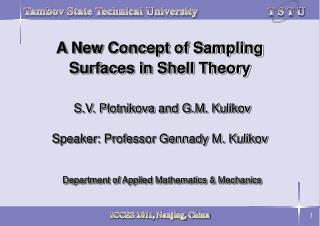Download PresentationKinematic Description of Undeformed Shell# Kinematic Description of Undeformed Shell - PowerPoint PPT Presentation

Download Presentation##### Kinematic Description of Undeformed Shell

Download Policy: Content on the Website is provided to you AS IS for your information and personal use and may not be sold / licensed / shared on other websites without getting consent from its author. While downloading, if for some reason you are not able to download a presentation, the publisher may have deleted the file from their server.

- - - - - - - - - - - - - - - - - - - - - - - - - - - E N D - - - - - - - - - - - - - - - - - - - - - - - - - - -
##### Presentation Transcript

1. A New Concept of Sampling Surfaces in Shell TheoryS.V. PlotnikovaandG.M. KulikovSpeaker: Professor Gennady M. KulikovDepartment of Applied Mathematics & Mechanics

2. 1, 2, …, N- sampling surfaces (S-surfaces) r(1, 2) - position vector of midsurface  R = r+3e3 - position vectors of S-surfaces I = 1, 2, …, N I I I Figure 1. Geometry of the shell Base Vectors of Midsurface and S-Surfaces (1) (2) ei - orthonormal vectors;A, k -Lamé coefficients and principal curvatures of midsurface c = 1+k3 - shifter tensor at S-surfaces;3- transverse coordinates of S-surfaces (I = 1, 2, …, N) I I I Kinematic Description of Undeformed Shell

3. Position Vectors of Deformed S-Surfaces (3) (4) I u (1, 2) - displacement vectors of S-surfaces I = 1, 2, …, N Figure 2. Initial and current configurations of the shell Base Vectors of DeformedS-Surfaces I  (1, 2) - derivatives of 3D displacement vector at S-surfaces (I = 1, 2, …, N) Kinematic Description of Deformed Shell

4. (5) (6) (7) Green-Lagrange Strain Tensor at S-SurfacesLinearized Strain-Displacement RelationshipsRepresentation for Displacement Vectors in Surface Frame

5. (8) (9) (10) Remark 1. Strains (10) exactly represent all rigid-body shell motions in any convected curvilinear coordinate system Representation for Derivatives of Displacement VectorsStrain ParametersStrains of S-Surfaces

6. Higher-Order Shell Theory (11) (12) (13) (14) I L (3) -Lagrange polynomials of degree N - 1(I = 1, 2, …, N) Displacement Distribution in Thickness DirectionPresentation for Derivatives of 3D Displacement Vector Strain Distribution in Thickness Direction

7. (15) (16) (17) (18) (19) - + pi , pi - surface loads acting on bottom and top surfaces Remark 2. It is possible to carry out exact integration in (19) using the n-point Gaussian quadrature rule with n = N+1 Stress ResultantsVariational EquationConstitutive EquationsPresentation for Stress Resultants

8. (20) (21) Nr (1, 2)- bilinear shape functions  = (- c)/ - normalized coordinates Figure 3. Biunit square in (1, 2)-space mapped into the exact geometry four-node shell element in (x1, x2, x3)-space Displacement InterpolationAssumed Strain Interpolation Finite Element Formulation

9. Numerical Examples 1. Square Plate under Sinusoidal Loading Figure 4. Simply supported square plate with a = b =1 , E = 107 and  = 0.3 Table 1. Results for a thick square plate with a / h = 2

10. Table 2. Results for thick and thin square plates with five equally located S-surfaces Figure 5. Distribution of stresses S13 and S33 through the plate thickness: Vlasov’s solution ( ) and present higher-order shell theory for N = 7 ( )

11. 2. Cylindrical Composite Shell under Sinusoidal Loading Figure 6. Simply supported cylindrical composite shell (modeled by 32128 mesh) Table 3. Results for a thick cylindrical shell with R / h = 2

12. Table 4. Results for thick and thin cylindrical shells with seven S-surfaces Figure 7. Distribution of stresses S33 through the shell thickness: exact solution ( ) and present higher-order shell theory for N = 7 ( )

13. 3. Spherical Shell under Inner Pressure Figure 8. Spherical shell under inner pressure with R = 10,  = 89.98, E = 107 and  = 0.3 (modeled by 641 mesh) Table 5. Results for a thick spherical shell with R / h = 2

14. Table 6. Results for thick and thin spherical shells with seven S-surfaces Figure 9. Distribution of stresses S11 and S33 through the shell thickness: Lamé’s solution ( ) and present higher-order shell theory for N = 7 ( )

15. Conclusions • A simple and efficient concept of S-surfaces inside the shell body has been proposed. This concept permits the use of 3D constitutive equations and leads for the sufficient number of S-surfaces to the numerically exact solutions of 3D elasticity problems for thick and thin shells • A new higher-order theory of shells has been developed which permits the use, in contrast with a classic shell theory, only displacement degrees of freedom • A robust exact geometry four-node solid-shell element has been built which allows the solution of 3D elasticity problems for thick and thin shells of arbitrary geometry